# KVPY-SA 2017 Physics Paper with Solutions

KVPY is an examination held to encourage students to pursue a career in science research. KVPY examination is conducted in subjects like Physics, Chemistry, Mathematics and Biology. KVPY-SA Physics question paper includes questions from chapters like optics, motion in one dimension, motion in two dimensions, fluid mechanics, etc. The questions are based on Class 10, Class 11 and Class 12 syllabus.

### KVPY SA 2017 - Physics

Question 1: Consider the following statements (X and Y stand for two different elements)

(I) 32X65 and 33Y65 are isotopes

(II) 42X86 and 42Y85 are isotopes.

(III) 85X174 and 88Y177 have the same number of neutrons.

(IV) 92X235 and 94Y235 are isobars

The correct statements are:

1. a. II and IV only
2. b. I, II and IV only
3. c. II, III and IV only
4. d. I, II, III and IV only

Solution:

Any element represents by

zXA

Where, x = elements symbol

A = mass number = sum of (Proton + Neutron)= P + n

Z = atomic number = No. of protons

Isotopes: Number of protons same

Isobars: mass number same

Since, We can write also,

No. of neutrons = A – Z ⇒ n

Since, 85X174 ⇒ n = 174 – 85 = 89

88Y177 ⇒ n = 177 – 88 = 89

Question 2: A student performs an experiment to determine the acceleration due to gravity g. The student throws a steel ball up with initial velocity u and measures the height h travelled by it at different times t. The graph the student should plot on a graph paper to readily obtain the value of g is

1. a. h versus t.
2. b. h versus t2
3. c. h versus $\sqrt{2}$
4. d. (h/t) versus t

Solution: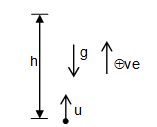By applying 2nd equation of motion:

$s=ut+\frac{1}{2}at^{2}$

$h=ut+\frac{1}{2}(-g)t^{2}$

$h=ut-\frac{1}{2}(g)t^{2}\Rightarrow \: parabola$

For readily obtain the value of g, graph should be straight line not parabola.

So, dividing by t on both side

$\frac{h}{t}=u-\frac{g}{2}t$

Let (h/t) = y, t=x

And compare it with standard equation of straight line.

y = mx + C

So, m = (-g/2) and c = u

⇒ g = –2m

Now the student can readily obtain the value of g.

Question 3: A person goes from point P to point Q covering 1/3 of the distance with speed 10km/hr, the next 1/3 of the distance at 20 km/hr and the last 1/3 of the distance at 60 km/hr. The average speed of the person is

1. a. 30 km/hr
2. b. 24 km/hr
3. c. 18 km/hr
4. d. 12 km/hr

Solution: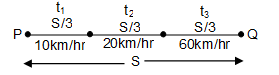Average speed = total distance covered/time taken

$=\frac{S}{t_{1}+t_{2}+t_{3}}$

$Speed=\frac{distance}{time}\Rightarrow time=\frac{distance}{speed}$

$t_{1}=\frac{S/3}{10},t_{2}=\frac{S/3}{20},t_{3}=\frac{S/3}{60}$

Put the values of t1, t2 and t3 in formula of average speed

$v_{Avg}=\frac{S}{\frac{S}{3}\left [ \frac{1}{10}+\frac{1}{20}+\frac{1}{60} \right ]}=\frac{3}{\frac{1}{10}+\frac{1}{20}+\frac{1}{60}}$

$=\frac{3}{\frac{6+3+1}{60}}=\frac{30\times 60}{10}=18 km/hr$

Question 4: A person looks at the image of two parallel finite length lines PQ and RS in a convex mirror (see figure).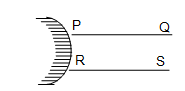Which of the following represents schematically the image correctly? (Note: Letters P, Q, R and S are used only to denote the endpoints of the lines.)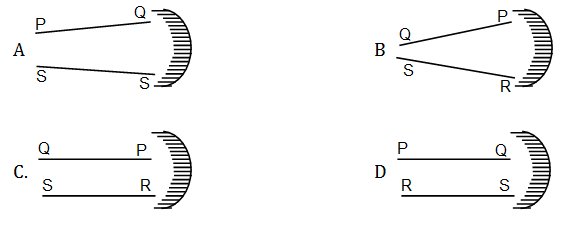Solution:

When an object is placed in front of convex mirror then the image formed by it is virtual, in front of mirror, erect, smaller in size.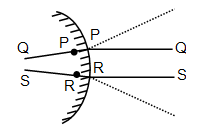Question 5: In Guericke’s experiment to show the effect of atmospheric pressure, two copper hemispheres were tightly fitted to each other to form a hollow sphere and the air from the sphere was pumped out to create vacuum inside. If the radius of each hemisphere is R and the atmospheric pressure is P, then the minimum force required (when the two hemispheres are pulled apart by the same force) to separate the hemispheres is

1. a. 2 πR2P
2. b. 4 πR2P
3. c. πR2P
4. d. πR2P/2

Solution: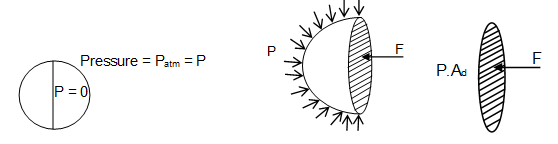Where,

F = Force

P = Pressure

Patm = Atmospheric pressure

Ad = Projected area of disc

Therefore, the minimum force required to separate the hemisphere is

= P. πR2

F = πR2. P

Question 6: Positive point charges are placed at the vertices of a star shape as shown in the figure. Direction of the electrostatic force on a negative point charge at the centre O of the star is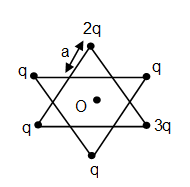1. a. towards right
2. b. vertically up
3. c. towards left
4. d. vertically down

Solution: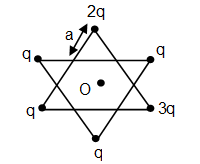Let distance of each positive charge from O = r

Use the formula of F = $=\frac{Kq_{1}q_{2}}{r^{2}}$

Force between q and –Q charge

$F_{q}=\frac{KqQ}{r^{2}}$

Force between 2q and –Q charge

$F_{2q}=\frac{Kq\times 2\times Q}{r^{2}}=2F_{q}$

Force between 3q and –Q charge.

$F_{3q}=\frac{Kq\times 3\times Q}{r^{2}}=3F_{q}$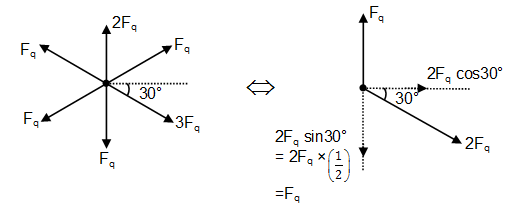Now, 2Fq cos 30°

$2F_{q}\times \frac{\sqrt{3}}{2}=\sqrt{3}F_{q}=\sqrt{3}\frac{KqQ}{r^{2}}$

Hence, direction of the electrostatic force is in horizontal direction towards right.

Question 7: A total solar eclipse is observed from the earth. At the same time an observer on the moon views the earth. She is most likely to see (E denotes the earth)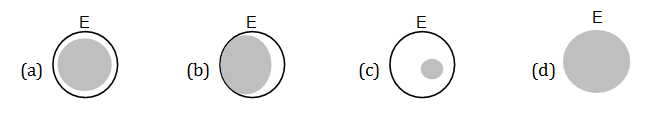1. a. a
2. b. b
3. c. c
4. d. d

Solution:

Earth revolves around sun and moon revolves around earth.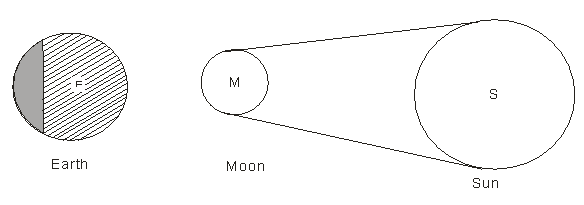The above figure represents the solar eclipse. As we can see here that Moon blocks some of Sun rays to reach on the small part of Earth and thus Moon forms a shadow on Earth during solar eclipse. So, a person on Moon sees a shadow of Moon on Earth.

Question 8: Ice in a freezer is at –7°C. 100 g of this ice is mixed with 200 g of water at 15°C. Take the freezing temperature of water to be 0°C, the specific heat of ice equal to 2.2 J/g °C, specific heat of water equal to 4.2 J/g °C, and the latent heat of ice equal to 335 J/g. Assuming no loss of heat to the environment, the mass of ice in the final mixture is closest to

1. a. 88 g
2. b. 67g
3. c. 54 g
4. d. 45 g

Solution: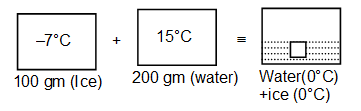Heat given by water = mc ΔT

(Here, m = mass, c = specific heat & ΔT = change in temperature.

= 200 × 4.2 × 15 J

Let m gram of ice melted.

Heat absorbed by ice = 100 × 2.2 × 7 + m + 335

100 × 2.2 × 7 + m × 335 = 200 × 4.2 × 15

m = 33 gm

Ice remained in final mixture = 100 – 33 gm = 67 gm

Question 9: A point source of light is placed at 2f from a converging lens of focal length f. A flat mirror is placed on the other side of the lens at a distance d such that rays reflected from the mirror are parallel after passing through the lens again. If f = 30 cm, then d is equal to

1. a. 15 cm
2. b. 30 cm
3. c. 45 cm
4. d. 75 cm

Solution: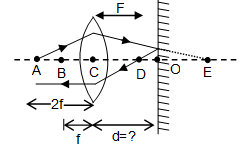For object placed at centre of curvature of lens (2f) image is formed on the other side of lens at centre of curvature.

Hence,

CE = 2f

CD = f

CE – CD = DE = 2f – f = f

Due to Reflection or its laws

OD = OE

OD + OE = DE = 20D = f

$\Rightarrow OD< \frac{f}{2}$

$d=CO=CD+OD=f+\frac{f}{2}=\frac{3f}{2}$

d=(3/2) x 30

d=45 cm

Question 10: The word ‘’KVPY’’ is written on a board and viewed through different lenses such that board is at a distance beyond the focal length of the lens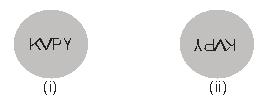Ignorging magnification effects, consider the following statements

(I) Image (i) has been viewed from the planar side of a plano-convex lens and image (ii) from the convex side of a plano-convex lens.

(II) Image (i) has been viewed from the concave side of a plano-concave lens and image (ii) from the convex side of a plano-convex lens.

(III) Image (i) has been viewed from the concave side of a plano-concave lens and image (ii) from the planar side of a plano-convex lens.

(IV) Image (i) has been viewed from the planar side of a plano-concave lens and image (ii) from the convex side of a plano-convex lens.

Which of the above statements are correct?

1. a. All four
2. b. Only (III).
3. c. Only (IV).
4. d. Only (II), (III) and (IV).

Solution:

Plano-concave lens:- If object is beyond focal length then image will be erected (straight).

Plano-convex lens:- If object is beyond focal length then image will be inverted.

Question 11: A ball is dropped vertically from height h and is bouncing elastically on the floor (see figure). Which of the following plots best depict the acceleration of the ball as a function of time.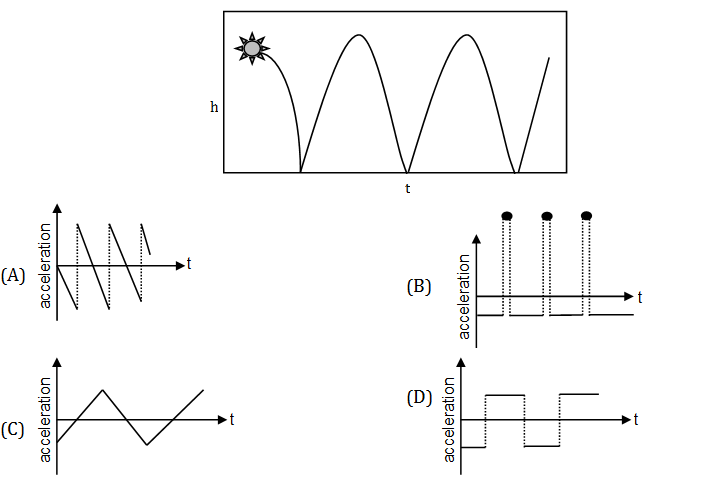Solution: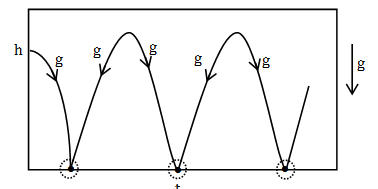At the point of collision an impact force will act and which is of impulsive nature. So at the time of impact since time of impact is very less. So, the impact force will increase. Thus, apart from gravitational acceleration, a force also will act and the value of gravitational acceleration will increase so high for a short time. The value of gravitational acceleration is negative because g is in downward direction.

Question 12: A student studying the similarities and differences between a camera and the human eye makes the following observations.

(I) both the eye and the camera have convex lenses.

(II) In order to focus, the eye lens expands or contracts while the camera lens moves forward or backward.

(III) The camera lens produces upside down real images while the eye lens produces only upright real image.

(IV) A screen in camera is equivalent to the retina in the eyes.

(V) A camera adjusts the amount of light entering in it by adjusting the aperture of the lens. In the eye the cornea controls the amount of light

The correct statements are:

1. a. Only (I), (II) (IV)
2. b. Only (I), (III), (V)
3. c. Only (I), (II), (IV), (V)
4. d. All

Solution:

Statement (I) and (II) are correct that both human eye and camera have convex lenses and the eye lens either contracts or expands in order to focus the image with the help of iris and camera lens moves forward or backward to focus the image.

Statement (III) is incorrect because eye lens produces inverted image on retina.

Statement (IV) is correct that retina in the eye acts as screen on which image is formed.

Statement (V) is incorrect because amount of light entering in the eye is controlled by pupil.

Question 13: A particle starts moving along a line from zero initial velocity and comes to rest after moving distance d. During its motion it had a constant acceleration f over 2/3 of the distance, and covered the rest of the distance with constant retardation. The time taken to cover the distance is

1. a. $\sqrt{2d/3f}$
2. b. $2\sqrt{d/3f}$
3. c. $\sqrt{3d/f}$
4. d. $\sqrt{3d/2f}$

Solution: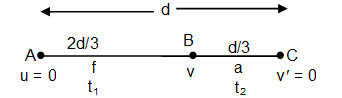Let velocity of B be v from A to B

Velocity at B, v = 0 + ft1 = ft1 ………(1)

Also distance covered, $\frac{2d}{3}=0+\frac{1}{2}ft_{1}^{2}$

$\Rightarrow t_{1}=\sqrt{\frac{4d}{3f}}$ --------(b)

From B to C:

Using, v’ = v – at2

O = v –at2 Þ v = at2 ……..(2)

From (1) and (2), we get a = ft1/t2

Distance covered, $\frac{d}{3}=\frac{v^{2}-v'^{2}}{2a}$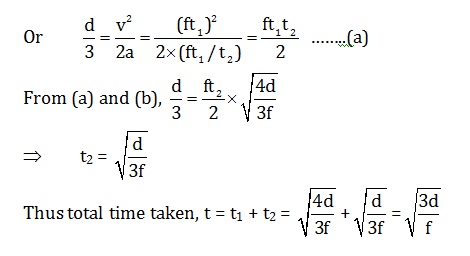Question 14: If the image formed by a thin convex lens of power P has magnification m then image distance υ is

1. a. $\nu =\frac{1-m}{P}$
2. b. $\nu =\frac{1+m}{P}$
3. c. $\nu =\frac{m}{P}$
4. d. $\nu =\frac{1+2m}{P}$

Solution:

Using lens formula:

$\frac{1}{v}-\frac{1}{u}=\frac{1}{f}$

Where, u = object distance, v = image distance & f = focal length

Where focal length of the lens f = 1/P

So, we get, $\frac{1}{v}-\frac{1}{u}=P$

Or 1 – (v/u) =Pv

Also, magnification of lens m = v/u

1 – m = Pv

$\Rightarrow v=\frac{1-m}{P}$

Question 15: A long cylindrical pipe of radius 20 cm is closed at its upper end and has an airtight piston of negligible mass as shown. When a 50 Kg mass is attached to the other end of the piston, it moves down. If the air in the enclosure is cooled from temperature T to T – ΔT, the piston moves back to its original position. Then ΔT/T is close to (Assuming air to be an ideal gas, g = 10 m/s2, atmospheric pressure is 105 Pascal),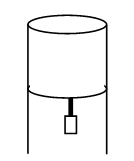1. a. 0.01
2. b. 0.02
3. c. 0.04
4. d. 0.09

Solution: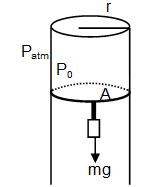Let the initial pressure = P0

Radius of the pipe = 20 cm = 0.2

g = 10 m/s2

Patm = 105 Pa

When 50 kg mass is suspended

Net pressure = initial pressure – Pressure force due to weight

$=\left ( P_{0}-\frac{mg}{A} \right )$

Temperature is constant and PV = nRT.

PiVi = PfVf

Put the value of Pf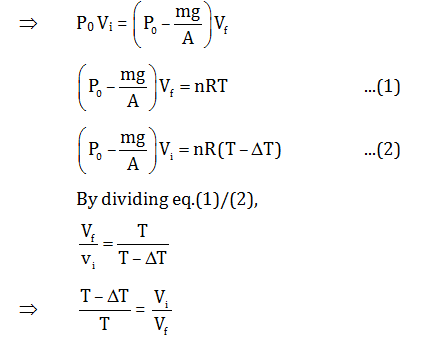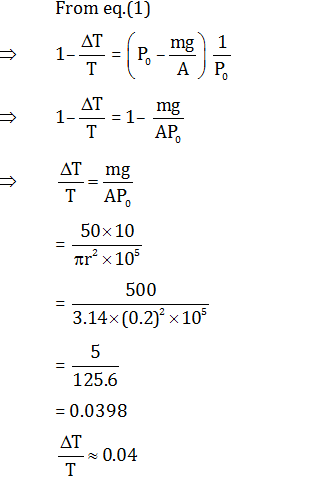Question 16: Two different liquids of same mass are kept in two identical vessels, which are placed in a freezer that extracts heat from them at the same rate causing each liquid to transform into a solid. The schematic figure below shows the temperature T vs time t plot for the two materials. We denote the specific heat in the liquid status to be CL1 and CL2 for materials 1 and 2 respectively, and latent heats of fusion U1 and U2 respectively.

Choose the correct option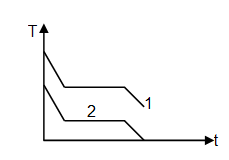1. a. CL1 > CL2 and U1 < U2
2. b. CL1 > CL2 and U1 > U2
3. c. CL1 < CL2 and U1 > U2
4. d. CL1 < CL2 and U1 < U2

Solution:Rate of heat extracted

$R=\frac{-mc_{L}\Delta T}{\Delta T}\Rightarrow \Delta T=\frac{-R}{mc_{L}}\Delta T$

Since, magnitude of slope of liquid 1 is greater than that of liquid 2.

So, $\frac{R}{mc_{L_{1}}}> \frac{R}{mc_{L_{2}}}$

Where m and R are constants

$C_{L_{2}}> C_{L_{1}}$

Part of graph where T is constant represents the phase change of substance. Since, more time is required for liquid 1 to change into solid. So more heat is extracted from liquid 1 as compared to liquid 2. Where heat extracted H = mV

$\Rightarrow$ U1 > U2 (Since H1 > H2)

Question 17: A long horizontal mirror is next to a vertical screen (See figure). Parallel light rays are falling on the mirror at an angle α from the vertical. If a vertical object of height h is kept on the mirror at a distance d > h tan (α). The length of the shadow of the object on the screen would be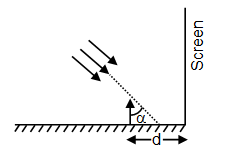1. a. h/2
2. b. h tan (α)
3. c. 2h
4. d. 4h

Solution: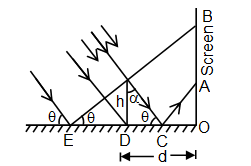It can be concluded from the attached ray diagram that on the screen, portion AB will be shadow on the screen

From laws of reflection

∠OCA = θ

∠OCA = 90° – α

OA = OC tan θ

OA = (OD – CD) cot α

OA = (d – h tan α) cot α

OA = d cot α – h ……..(1)

OB = OE tan θ

OB = (OD + ED) tan θ

OB = (d + h cot θ) tan θ

OB = d cot α + h ……(2)

Length of shadow, AB = OB – OA = 2h

Question 18: A spherical marble of radius 1 cm is stuck in a circular hole of radius slightly smaller than its own radius (for calculation purpose, both can be taken same) at the bottom of a bucket of height 40 cm and filled with water up to 10 cm. If the mass of the marble is 20 g, the net force on the marble due to water is close to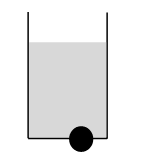1. a. 0.02 N upward
2. b. 0.02 N downward
3. c. 0.04 N upward
4. d. 0.04 N downward

Solution: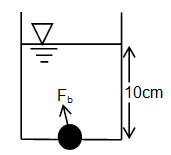A buoyancy force due to water will be on half part (which is in water) of the marble.

If Fb is the buoyancy force and Fp is the pressure force due to water column then the net force Fnet which is downward is,

Fnet = Fp – Fb

$=(\delta gH)A_{p}-\delta _{liquid}V_{object}G$

Since, $A_{p}\rightarrow Projected Area (\pi R^{2})$

Therefore, $F_{net}=\delta gHA_{p}-\delta \frac{V}{2}.g$

$=10^{3}\times 10\times 10\times 10^{-2}\times (\pi \times (10^{-2})^{2})-10^{3}\times \frac{2}{3}\pi (10^{-2})^{3}\times 10$

Since, $V=\frac{4}{3}\pi R^{3}\Rightarrow \frac{V}{2}=\frac{2}{3}\pi R^{3}$

Fnet = 0.04

And the direction is downward.

Question 19: In the circuit shown below (on the left) the resistance and the emf source are both variable. The graph of seven readings of the voltmeter and the ammeter (V and I, respectively) for different setting of resistance and the emf, taken at equal intervals of time Δt, are shown (on the right) by the dots connected by the curve EFGH. Consider the interval resistance of the battery to be negligible and the voltmeter and ammeter to be ideal devices. Take R0 = V0/I0.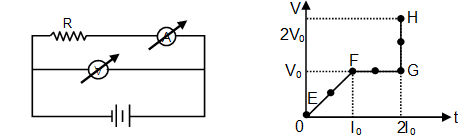Then the plot of the resistance as a function of time corresponding to the curve EFGH is given by.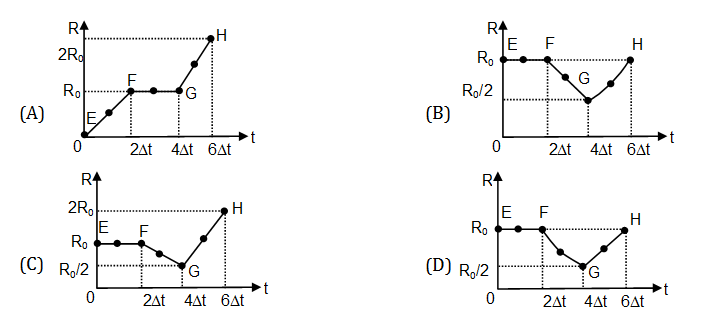Solution:

Resistance, R = V/I

Region E to F:

Slope of V – I curve is constant, thus resistance is constant.

Resistance REF = V0/I0 =R0

Region F to G:

Voltage is constant i.e. V = constant

$\Rightarrow R\propto \frac{1}{I}$

So, resistance decreases in a hyperbolic manner with increase in voltage increase in current

Resistance at G, $R_{G}=\frac{V_{0}}{2I_{0}}=R_{0}/2$

Region G to H:

Current is constant i.e. I = constant

$\Rightarrow R\propto V$

So, resistance increases linearly with increase in voltage.

Resistance at H, $R_{H}=\frac{2V_{0}}{2I_{0}}=R_{0}$

Question 20: Stoke’s law states that the viscous drag force F experienced by a sphere of radius a, moving with a speed V through a fluid with coefficient of viscosity η, is given by F = 6 πnav. If this fluid is flowing through a cylindrical pipe of radius r, length l and a pressure difference of P across its two ends, then the volume of water V which flows through the pipe in time t can be written as $\frac{V}{t}=k\left [ \frac{P}{l} \right ]^{a}\eta ^{b}r^{c}$

where k is a dimensional constant. Correct values of a, b and c are

1. a. a = 1, b = –1, c = 4
2. b. a = –1, b = 1, c = 4
3. c. a = 2, b = –1, c = 3
4. d. a = 1, b = –2, c = –4

Solution:

Given relation

$\frac{V}{t}=k\left [ \frac{P}{l} \right ]^{a}\eta ^{b}r^{c}$

Where V $\rightarrow$ volume,

t $\rightarrow$ time,

k $\rightarrow$constant

P $\rightarrow$pressure,

l $\rightarrow$length

η $\rightarrow$ coefficient of viscosity

R $\rightarrow$ radius

Now equate the dimension of both the sides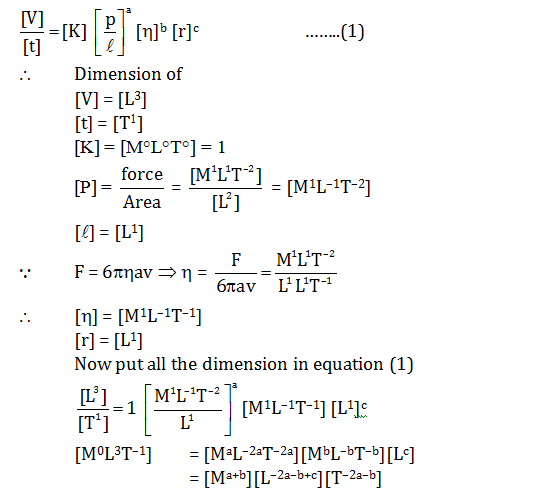Now compare powers of both the sides,

a + b = 0 …..(2)

– 2a – b + c = 3 …..(3)

–2a – b = –1 …..(4)

From equation (3) and (4)

c = –4

⇒ a = – b

∴ –2a + a = – 1

a = 1

b = –1

So,a, b, c are 1, –1, –4 respectively

### KVPY-SA 2017 Physics Paper with Solutions